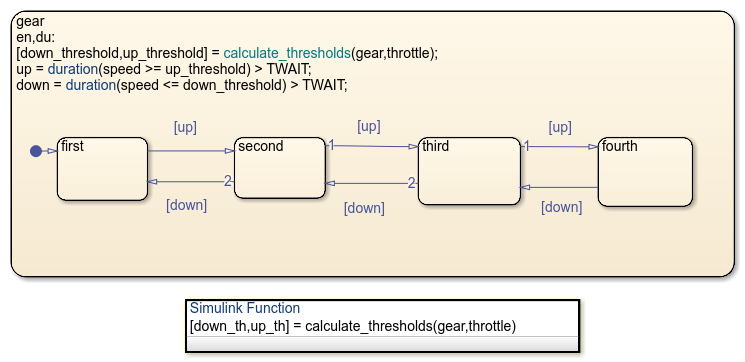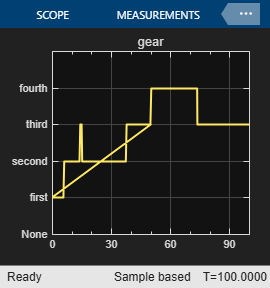# Implement an Automatic Transmission Gear System by Using the `duration` Operator

This example models an automotive transmission system by using the Stateflow® temporal logic operator `duration` to automatically shift gears based on the vehicle throttle requirements and speed. For more information, see Control Chart Execution by Using Temporal Logic.

### Model Description

There are five major blocks in this model.

• User Inputs: Provides two inputs to the model, brake and throttle.

• Engine: Calculates engine RPM based on impeller torque value and throttle.

• Gear_logic: Calculates next gear based on current gear, throttle, and current vehicle speed.

• Transmission: Calculates impeller and output torque based on RPM, gear and transmission speed.

• Vehicle: Calculates vehicle and transmission speed based on output torque and brake.### Chart Description

The Stateflow chart models the shifting of gears based on throttle and speed of the vehicle. The `down_threshold` and `up_threshold` outputs represent minimum and maximum speed values that throttle and current gear are able to handle. The Simulink function `calculate_thresholds` calculates these two values using `throttle` and `gear` as inputs. If the actual speed is higher than `up_threshold` for longer than `TWAIT`, then the chart transitions to higher gear. Conversely, if the actual speed is lower than `down_threshold` for longer than `TWAIT`, then the chart transitions to a lower gear. At each time step, the chart calls the `duration` operator to find the amount of time for which `speed` is higher than `up_threshold`. If this time exceeds `TWAIT` then boolean variable `up` is set which in turn transitions chart from current gear to a higher gear. Conversely the chart transitions to a lower gear based on the value of `down_threshold`.### Active State Data

Active State Data is the enumerated data that represents the current active state during simulation. In this chart, the output data `gear` maintains the current active state which in turn represents the current gear. This data automatically updates when a transition is taken. The data is used by downstream blocks as well as by the Simulink® function `calculate_thresholds`. For more information, see Monitor State Activity Through Active State Data.

### Simulation

To visualize these changes, simulate the model and open the scope.